# How To Solve Circular Seating Arrangement Problems – Tips And Tricks

Circular Seating Arrangement Puzzles are repeatedly asked in every Banking and Insurance exams conducted by IBPS. Puzzles are time consuming questions and mostly the candidates lose their marks due to reasoning section. Most of the candidates do not even dare to solve these questions and they prefer to just solve the Statement and Conclusion kind of questions to just anyhow make it to the cut offs of the reasoning. In fact the candidates who all are getting selected in various exams are good at these kinds of puzzles. So why can’t we solve these puzzles ? Because of constant fear of losing time . But once you solve these problems you could easily answer questions. If you practice hard these puzzles, it may not take you more than 4-5 minutes for answering 5 questions and easy 5 marks. Let’s give some tips and tricks to solve these puzzles easily and in time efficient manner.

Rules of Circular Seating Arrangement Problems :

1. When Facing Towards The Center :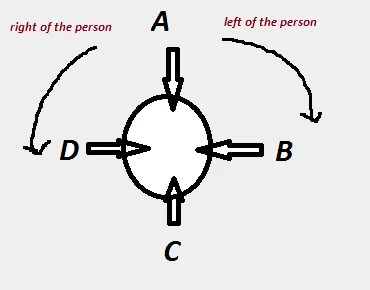Person Sitting Left To A Person : Clockwise Direction (As given in the picture , B is sitting Left to A)

Person Sitting Right to A Person : Anti-Clockwise Direction ( As given in the picture , D is sitting Right to A)

2. When Facing Away From The Center :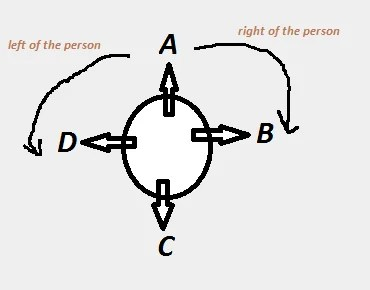Person Sitting Left To A Person :  Anti -Clockwise Direction (As given in the picture , D is sitting Left to A)

Person Sitting Right to A Person : Clockwise Direction ( As given in the picture , B is sitting Right to A)

How To Solve These Problems :

Lets take an example and then we will solve it with all the methods to be use. Just remember never give up these problems, its all about having a fresh mind and solving techniques. Read the question carefully.

Example 1 : P , Q , R , S, T , U , V and W are eight friends sitting around a circle facing towards the center.

• W is on the immediate left of P but is not the neighbour of T or S
• U is on the immediate right of Q and V is the neighbour of T.
• R is between T and U.

Solution 1 :  Since the problem above says that All are facing towards the center, so remember the first rule given above (i.e. left is clockwise , right is anti-clockwise)

Now separately solve the problem using data,

So the first given data is W is on the immediate left of P but is not the neighbour of T or S , So the diagram would be like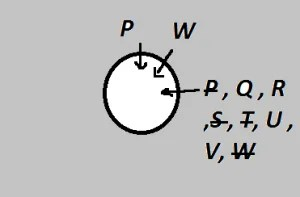So Now we know  Only Q ,R, U, V could be immediate left of W

Now lets move to second data , U is on the immediate right of Q and V is the neighbour of T ,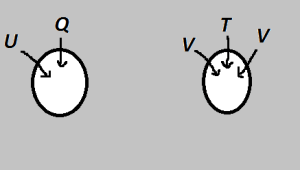So Lets move to the third data, R is between T and U ,

Actually You cannot understand the problem like this, that’s why you have to go with one diagram and solve it using all the possible values and eliminations.

So Now lets solve the problem from all the data with condition 1 diagram ,Here comes the game of logic , As you notice that R is between T and U , So now we can eliminate the possibility of R from being the neighbour (immediate left) of W.

So now we remain with Q , U , V as the option left. It could not be Q because immediate right of Q is U but here it would be W which is not possible. So we will eliminate Q.

So now we remain with U , V as the option left. Suppose it is U, then Q would be at immediate left of it which is not possible because R is between T and U not Q. Therefore possibility of Q is also eliminated.

Therefore V is the immediate left of W.

Since T is the neighbour of V, So now T would be at immediate left of V.

Since R is between T and U, R would be at the immediate left of T and U would be at the immediate left of R.

Since U is the immediate right of Q, therefore Q would be placed at the immediate left of U.

Now there is only one vacant position remaining that would be taken by S which is immediate right of P and immediate left of Q.

So now the final diagram would be like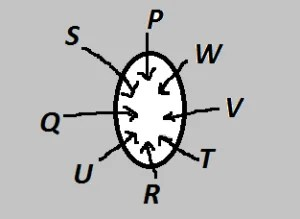So what we understood that we need to solve the problems by assuming the places and eliminating the non possibilities and go with the conditions given in the problem. Most important part is remembering the rule of towards the center and away from the center. The given problem would not take more than 4 minutes to solve and you can easily get full marks for this kind of problems. Let’s solve questions based on the given problem :

Question 1 : What is the position of S ?

1.  On the immediate left of Q
2. Second to the right of Q
3. Between Q and U
4. On the immediate left of P
5. None of These

Answer 1 : As from the final diagram, we can infer that S is on the immediate left of Q. So the answer is 1.

Question 2 : Which of the following statements is true ?

1. T is between U and Q
2. U is the neighbour of V
3. V is between W and T
4. W is between P and S
5. None of These

Answer 2 : As from the final diagram, we can infer that V is between W and T is the right answer. So Answer is 3.

This site uses Akismet to reduce spam. Learn how your comment data is processed.